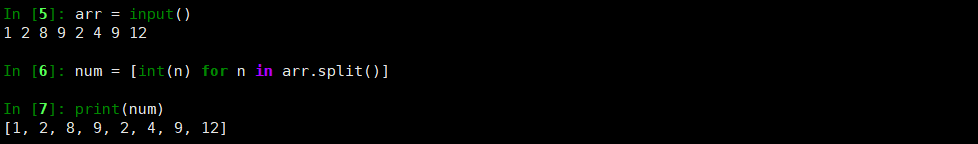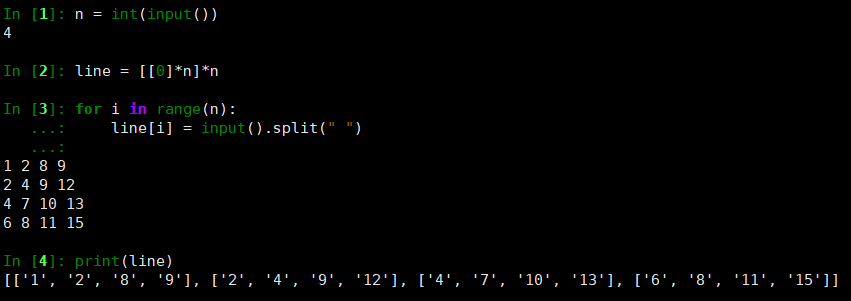• 下面小编就为大家分享一篇Python输入二维数组方法，具有很好的参考价值，希望对大家有所帮助。一起跟随小编过来看看吧
• Python中创建二维数组 Python中的列表list可以当做一维数组使用，但是没有直接的定义使用二维数组。如果直接使用a = [][]会产生SyntaxError: invalid syntax语法不正确错误。 一般Python中创建二维数组的方法是使用...
• ## Python输入二维数组

千次阅读 2017-02-21 15:16:50
前不久对于Python输入二维数组有些不解，今日成功尝试，记以备忘。这里以输入1-9,3*3矩阵为例n=int(input()) line=[*n]*n for i in range(n): line[i]=input().split(' ') print(line)使用数据转换为int即可
前不久对于Python输入二维数组有些不解，今日成功尝试，记以备忘。这里以输入1-9,3*3矩阵为例
n=int(input())
line=[*n]*n
for i in range(n):
line[i]=input().split(' ')
print(line)
使用数据转换为int即可
展开全文• 请完成一个函数，输入这样的一个二维数组和一个整数，判断数组中是否有该整数。 方法一： #时间复杂度 O(mn) class Solution: def find(self, target, array): for i in range(len(array)): for j in range(len...
• 一维数组： arr = input("") //输入一个一维数组，每个数之间使空格隔开 num = [int(n) for n in arr.split()] //将输入每个数以空格键隔开做成数组 ...（以n*n的二维数组为例） n = int(input()) /...
一维数组：
arr = input("")    //输入一个一维数组，每个数之间使空格隔开
num = [int(n) for n in arr.split()]    //将输入每个数以空格键隔开做成数组
print(num)        //打印数组
一维数组输入输出示例：二维数组:
（以n*n的二维数组为例）
n = int(input())        //输入二维数组的行数和列数
line = [*n]*n        //初始化二维数组
for i in range(n):
line[i] = input().split(" ")       //输入二维数组，同行数字用空格分隔，不同行则用回车换行
print(line)            //打印二维数组
二维数组打印示例：如果二维数组中的元素都为整型，可加上：
line[i] = [int(j) for j in line[i]]
即输入：
n = int(input())        //输入二维数组的行数和列数
line = [*n]*n        //初始化二维数组
for i in range(n):
line[i] = input().split(" ")       //输入二维数组，同行数字用空格分隔，不同行则用回车换行
line[i] = [int(j) for j in line[i]]    //将数组中的每一行转换成整型
print(line)            //打印二维数组

展开全文• 注意append里面是两层括号，这个非常重要，如果漏掉了就不是二维数组了，用axis的时候就会报维度不匹配。 以上这篇numpy向空的二维数组中添加元素的方法就是小编分享给大家的全部内容了，希望能给大家一个参考，也...
• 因一次笔试中忘记如何用python创建二维数组，遂记录下来. 成功没有捷径，一定要脚踏实地. 没有使用numpy模块，若想使用numpy模块创建二维数组请移步。 一：初始化一个元素从0 - n*m的二维数组 row = int(input...
因一次笔试中忘记如何用python创建二维数组，遂记录下来.
成功没有捷径，一定要脚踏实地.
没有使用numpy模块，若想使用numpy模块创建二维数组请移步。
一：初始化一个元素从0 - n*m的二维数组
row = int(input())
column = int(input())
dp = [[i*column + j for j in range(column) ] for i in range(row)]
#第i行第j列元素=元素所在行数*总列数+该元素所在的列数
print(dp)
二：初始化一个元素全为0的二维数组
row = int(input())
column = int(input())
dp = [[0 for j in range(column)] for i in range(row)]
print(dp)
三：手动输入，一个n*m的二维数组（一个一个元素输入）
row = int(input())
column = int(input())
dp = []
array = []
for i in range(row):
for j in range(column):
value = int(input())
array.append(value)
dp.append(array)
array = []
print(dp)
四：手动输入，一行一行输入
    '''
例：
输入 3
1 2
3 4
5 6
输出 [[1, 2], [3, 4], [5, 6]]
'''
row = int(input())
dp = []
for i in range(row):
#column = list(map(int, sys.stdin.readline().split(',')))  # 以,为分隔符
dp.append(column)
print(dp)
五：手动输入，一行输入全部数据
'''
例：
输入 1 2 3 4 5 6 7 8
4
2
输出 [[1, 2], [3, 4], [5, 6], [7, 8]]
'''

array = list(map(int,elements))
dp = []
row = int(input())
column = int(input())
for i in range(row):
dp.append(array[i*column:(i+1)*column])
print(dp)

展开全文• 新手刚学python，用的是python3.4,想通过input输入二维数组后程序用数组中的元素进行运算，运行时有错误代码ValueError: invalid literal for int() with base 10， 该怎么解决？如果不能直接输入的话，是否可以通过...
• 程序分析：利用双重for循环控制输入二维数组，再将a[i][i]累加后输出。 def two_dimensionalArray(self): '二维数组实现求三阶矩阵的对角线元素之和' sum = 0 matrix = [[0, 1, 0], [0, 21, 0], [0, 12, 0]] ...
• python输入维数组 使用int()进行强制类型转型 当输入内容不为数字时，不能转型，发生except跳出循环。 先声明data是一个list，将input_A一个个拼接（+）进去。 data = [] while True: try: input_A = int...
python输入一维数组 使用int()进行强制类型转型 当输入内容不为数字时，不能转型，发生except跳出循环。 先声明data是一个list，将input_A一个个拼接（+）进去。
data = []
while True:
try:
input_A = int(input("Input: "))
data +=[input_A]
except:
break
print(data)
print(type(data))
print(type(data))

运行代码，从键盘输入
E:\Anaconda3\python.exe D:/pycode/NowCooder/数组的输入.py
Input: 1
Input: 2
Input: 3
Input: ；  # 输入英文分号停止输入
[1, 2, 3]
<class 'list'>
<class 'int'>

python输入二维数组 正则表达式（r’[\D]’）会利用非数字的字符进行切割，因此数字之间插入什么都无所谓。
import re
data2D = []
while True:
userInput = input('Input:')          # 输入数组，用空格隔开即可
info = re.split(r'[\D]', userInput)  # 正则表达式分割
data = []   # 定义一维数组
try:
for number in info:
data += [int(number)]  # 一维数组加入数字
data2D += [data]  # 一维数组加入到二维中去
except:
break
print(data2D)

测试二维数组的输入 输入最后一行字符后，连续按两次Enter键结束输入
E:\Anaconda3\python.exe D:/pycode/NowCooder/数组的输入.py
Input:1 2 3
Input:2,3,4
Input:1[2[3[4
Input:9-8-3-4
Input:
[[1, 2, 3], [2, 3, 4], [1, 2, 3, 4], [9, 8, 3, 4]]

Process finished with exit code 0

在Input：后按 Enter 停止输入
E:\Anaconda3\python.exe D:/pycode/NowCooder/数组的输入.py
Input:1 2 3
Input:
[[1, 2, 3]]

使用re.split来分割字符串 re.split(r’[\D]’, s) 表示将字符串 s 按“非数字”分割成一个列表，保留数字，去除非数字字符。
import re
s = input()  # abcd12345ed125ss123456789
ss = re.split(r'\D', s)
sss = re.split(r'[\D]', s)
# print(max(ss, key=len))  # 123456789
print(ss)   # ['', '', '', '', '12345', '', '125', '', '123456789']
print(sss)  # ['', '', '', '', '12345', '', '125', '', '123456789']
data = []
for num in ss:
if num != '':
data += [int(num)]   # [ ] 不能省略
print(data)  # [12345, 125, 123456789]


https://www.jianshu.com/p/d177f14a7d44 正则表达式 https://www.cnblogs.com/tina-python/p/5508402.html

展开全文• ## Python 旋转二维数组

千次阅读 2018-07-05 20:33:40
故事要从前几个月刷剑指offer的时候说起，那时候遇到过一个关于二维数组的问题，问题如下 输入一个矩阵，按照从外向里以顺时针的顺序依次打印出每一个数字，例如，如果输入如下矩阵： 1 2 3 4 5 6 7 8 9 10 11 12...
• 二话不说上代码： import xlwt workbook = xlwt.Workbook() sheet = workbook.add_sheet("Sheet") for i in range(len(rows)): for j in range(len...这样就可以把rows这个二维数组写入到test.xls文件里面了 ...
• 目的：生成初始值位0的m*n的二维数组 1.使用list方法 arr = [[0 for i in range(n)] for j in range(m)] 2.使用Numpy模块 arr = numpy.zeros((m, n)) 误区： arr = [*n]*m 使用该方法生成的二维数组...
• n = int(input()) a = [] for _ in range(n): a.append(list(map(int, input().rstrip().split()))) for i in range(n): print(a[i])
• 本文实例讲述了Python实现求两个数组交集的方法。分享给大家供大家参考，具体如下： 一、题目 给定两个数组，编写一个函数来计算它们的交集。 例1： 输入: nums1 = [1,2,2,1], nums2 = [2,2] 输出: [2,2] 例2： ...
• 请完成一个函数，输入这样的一个二维数组 和一个整数，判断数组中是否含有该整数。 d ef find(target,array): if (len(array)==0 or len(array)==0): return ‘数组不能为空’ big = len(array) small = len...
• ## python构建二维数组

千次阅读 2018-01-06 22:34:05
python构建二维数组，一般都需要使用循环来构建，为什么不能用类似于[*10]*10构建一个10*10的数组呢? 程序员嘛用代码说话，看下面的例子: 在上面的代码中构建了一个test_example的二维数组，改变其中的test...
• 二维数组的表示方法： python原生：列表的列表 Numpy:ndarray Pandas:DataFrame 二维ndarray与Python List 1.ndarry性能更好 2.ndarray有mean()、std()、sum()等更多的跟数学计算相关的内置函数，作用于整个...Numpy
• 请完成一个函数，输入这样的一个二维数组和一个整数，判断数组中是否含有该整数。 解题思路： 通过循环遍历二维数组中所有元素值与target值进行比较（暴力破解，非常好理解，不过复杂度相对高一点，但是也能编译...算法
• 发现这个问题在于LeetCode写算法时明明和题解一模一样但结果就是不对，排查发现问题出在定义数组上 我们先来看两个数组： 两个数组看似完全一致，...所以[*3]*2并不能当做我们所熟知的二维数组，建议以后定义二维数据结构
• 下面小编就为大家分享一篇Python获取二维矩阵每列最大值的方法，具有很好的参考价值，希望对大家有所帮助。一起跟随小编过来看看吧
• 二维数组排序python二维数组自定义排序基础二维数组排序二维数组自定义排序 python二维数组自定义排序 基础二维数组排序 y = sorted(x, key = lambda x:(x,-x)) # 按照一维升序，二维降序 输入：[(264.0, 8, 0...
• 二维数组转为一维数组 已知a = [[4, 0, 1], [9, 2, 7], [8, 12, 90]] 列表推导式 [i for j in a for i in j] itertools from itertools import chain list(chain.from_iterable(a)) sum小技巧 sum(a, []) ...
• 二维数组输入 n=int(input()) a=[] for _ in range(n): a.append(list(map(int,input().split(' ')))) print(a) 一维数组的输入 ########一维数组的输入3 a=list(map(int,input().split(' '))) print(a) ...
• 1 判断边界条件，输入值判断 2 一定要找终止条件,这里的终止条件是只要有一个list为空就退出 """ def conn(list1, list2): # 合并俩个有序非空的list res = [] while list1 and list2: if list1 <= list2...
• 一、函数 np.delete(array,obj,axis) 二、函数的意思 array:需要处理的矩阵 obj:需要处理的位置，比如要删除的第一行或者第一行和第二...x=np.array[[1,2,3],[4,5,6],[7,8,9]] #二维数组数据 删除行（axis=0）： 二numpy
• ## Python新建三维数组并赋值

万次阅读 多人点赞 2019-10-09 16:33:35
如何将二维数组赋值到三维数组，且包含对每个参数的理解。 1. 如何建立三维数组 若我们需要建立3✖️3✖️3的矩阵y，可以直接调用numpy库，代码如下： import numpy as np #导入numpy库 y = np.ones((3,3,3)) #新建...多维矩阵 赋值
• 1 m = int(input()) 2 grid = [[] for i in range(m)] 3 for i in range(m): 4 line = input().split(' ') 5 for j in range(len(line)): 6 grid[i].append(int(line[j])) 转载于:https://......

# python输入二维数组python 订阅